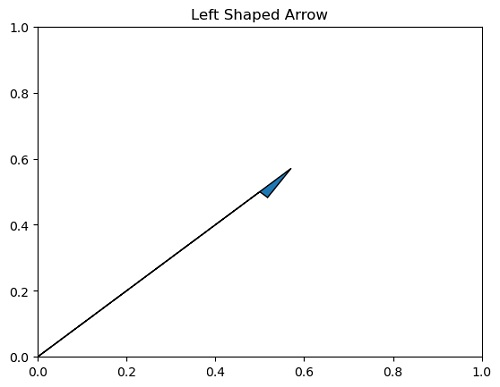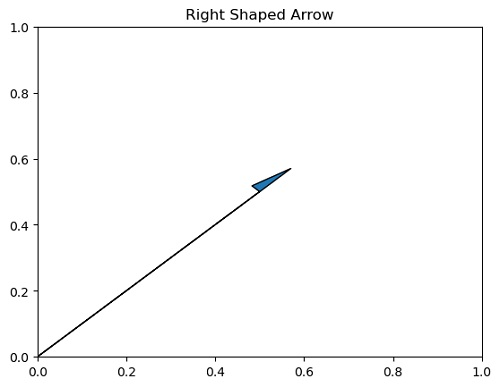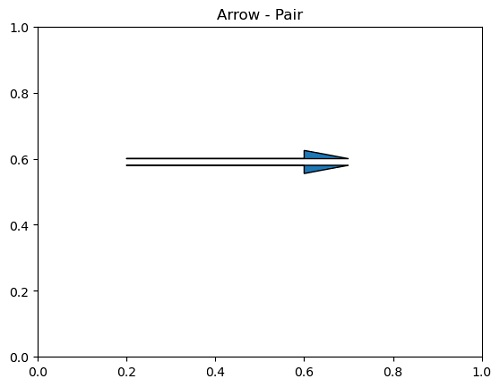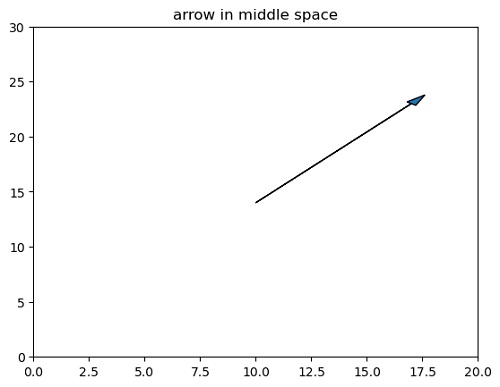# Right and Left Handed Arrows in Python Plot

matplotlib.pyplot.arrow(): Here, we are going to learn about the right and left handed arrows in Python with examples.
Submitted by Anuj Singh, on July 22, 2020

In this tutorial, we are going to explore two additional types of arrows that are Right Handed and Left Handed arrows. We can use the command shape in the function matplotlib.pyplot.arrow() for plotting Right and Left Handed arrows in a figure.

The following example illustrates the implementation.

```matplotlib.pyplot.arrow(
0,
0,
0.5,
0.5,

#shape = 'right' : Right Handed Arrow
#shape = 'left' : Left Handed Arrow
```## Code for Right and Left Handed Arrows in Python Plot

```import matplotlib.pyplot as plt

plt.figure()
plt.title('Left Shaped Arrow')
plt.show()

plt.figure()
plt.title('Right Shaped Arrow')
plt.show()

plt.figure()
plt.title('Arrow - Pair')
plt.show()
```

Output:

```Output is as figure
```

Languages: » C » C++ » C++ STL » Java » Data Structure » C#.Net » Android » Kotlin » SQL
Web Technologies: » PHP » Python » JavaScript » CSS » Ajax » Node.js » Web programming/HTML
Solved programs: » C » C++ » DS » Java » C#
Aptitude que. & ans.: » C » C++ » Java » DBMS
Interview que. & ans.: » C » Embedded C » Java » SEO » HR
CS Subjects: » CS Basics » O.S. » Networks » DBMS » Embedded Systems » Cloud Computing
» Machine learning » CS Organizations » Linux » DOS
More: » Articles » Puzzles » News/Updates